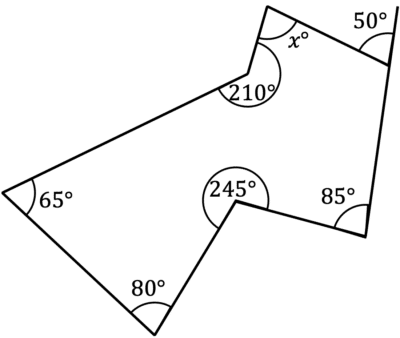# Interior and Exterior Angles

GCSELevel 4-5Level 6-7Cambridge iGCSE

## Interior and Exterior Angles

Interior angles are the angles inside a shape.

Exterior angles are formed when a line is extended from the vertex of a shape, and are the angles between the straight line and the shape.

You need to be able to identify and calculate interior and exterior angles of shapes with many different numbers of sides.

## Regular PolygonsRegular polygons have equal length sides, interior angles, and exterior angles.

We can calculate interior and exterior angles when we know the number of sides the regular polygon has:

Exterior angles: $\dfrac{360\degree}{\textcolor{green}{n}}$ where $\textcolor{green}{n}$ is the number of sides.

Interior angles: $180\degree -$ exterior angle size.Regular polygons can also be split into several isosceles triangles, from each side to the centre of the shape. The angle in the centre is always equal to the exterior angles.

Level 4-5GCSECambridge iGCSE

## ALL polygons – Regular and Irregular

The following rules are relevant for all shapes, whether they have equal angles (regular) or different angles (irregular).

Sum of all exterior angles in a shape: $360\degree$

Sum of all interior angles in a shape: $(\textcolor{green}{n}-2)\times 180\degree$

Level 6-7GCSECambridge iGCSE

## Example 1: Regular Polygons

Work out the size of each interior angle and each exterior angle of a regular octagon.

[4 marks]

With regular shapes, it is easiest to calculate the exterior angles first.

We can use the formula,

Exterior angle $= \dfrac{360\degree}{\textcolor{green}{n}}$

As we know octogons have $n=8$,

Exterior angle $= \dfrac{360\degree}{\textcolor{green}{8}}$

Exterior angle $= 45\degree$

Now we can calculate the size of each interior angle using,

Interior angle $= 180\degree -$ exterior angle size

Interior angle $= 180\degree - 45\degree$

Interior angle $= 135\degree$

Level 4-5GCSECambridge iGCSE

## Example 2: Irregular Polygons

Work out the size of angle $x$.[4 marks]

By counting the sides, we can see this shape is an irregular $7$ sided shape, or a heptagon.

As this shape is irregular, each angle is different, and we can use the following formula to work out the total size of the interior angles:

Sum of all interior angles $= (\textcolor{green}{n}-2)\times 180\degree$

Sum of all interior angles $= (\textcolor{green}{7}-2)\times 180\degree$

Sum of all interior angles $= 900\degree$

To work out angle $x$, we firstly need the interior angle adjacent to the exterior angle $50\degree$. As these lie on a straight line, they will add to $180\degree$, so the interior angle is $130\degree$.

We can now calculate $x$:

$900-130-85-245-80-65-210=85\degree$

Level 6-7GCSECambridge iGCSE

## Interior and Exterior Angles Example Questions

We know that $x$ will be equal to the exterior angles.

We can work out the exterior angles of a regular shape by:

$\dfrac{360\degree}{n}=\dfrac{360}{6}=60\degree$

If each interior angle is $140\degree$, each exterior angle must be $180\degree-140\degree=40\degree$.

As the shape is regular, we know that,

Exterior angle $=\dfrac{360\degree}{n}$

So,

$40\degree=\dfrac{360\degree}{n}\\$ $n=\dfrac{360\degree}{40}\\$ $n=9$

It is clear that this shape is irregular, so to find $x$, we can firstly find the exterior angle next to $x$, using:

Sum of exterior angles $=360\degree$

$360\degree-103\degree-96\degree-29\degree-78\degree=54\degree$

We can now work out the value of $x$:

$180-54=126\degree$

## You May Also Like...### MME Learning Portal

Online exams, practice questions and revision videos for every GCSE level 9-1 topic! No fees, no trial period, just totally free access to the UK’s best GCSE maths revision platform.

£0.00